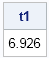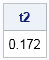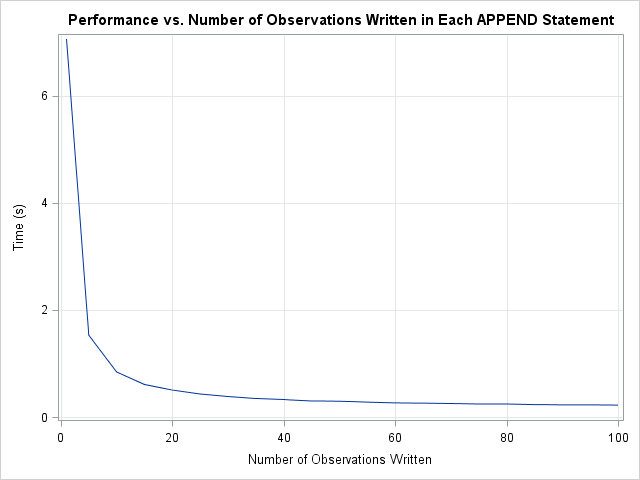I often blog about the usefulness of vectorization in the SAS/IML language. A one-sentence summary of vectorization is "execute a small number of statements that each analyze a lot of data." In general, for matrix languages (SAS/IML, MATLAB, R, ...) vectorization is more efficient than the alternative, which is to run many statements (often inside a loop) that each analyze a small amount of data.

I usually think of vectorization as applying to computations. However, I recently realized that the same principle applies when writing data to a SAS data set. I needed to compute a large number of results and write them to a SAS data set. Because each result required a similar computation, I wrote a program that looked something like the following.

```proc iml; N = 1e5; /* 100,000 = number of observations to write */ x = j(1,5);   t0 = time(); call randseed(123); create DS1 from x[colname=("x1":"x5")]; /* open data set for writing */ do i = 1 to N; call randgen(x, "Uniform"); /* replaces complex calculation */ append from x; /* write 1 obs with 5 variables */ end; close DS1; /* close data set */   t1 = time() - t0; print t1[F=5.3]; quit;```In the preceding program, the call to the RANDGEN function is used in place of a complicated computation. After running the program I was disappointed. Almost 7 seconds for a mere 100,000 results?! That's terrible performance! After studying the program I began to wonder whether the problem was that I was calling the APPEND statement many times, and each call does only a little bit of work (writes one observation). That is a classic vectorize-this-step situation.

I decided to see what would happen if I accumulated 1,000 results and then output those results in a single APPEND statement. My hope was that the performance would improve by calling the APPEND statement fewer times and writing more data with each call. The rewritten program looked similar to the following:

```proc iml; N = 1e5; /* total number of observations to write */ BlockSize = 1e3; /* number of observations in each APPEND stmt */ NumIters = N/BlockSize; /* number of calls to the APPEND stmt */ x = j(1,5);   t0 = time(); call randseed(123); xChunk = j(BlockSize,5,0); create DS2 from xChunk[colname=("x1":"x5")]; do i = 1 to NumIters; do j = 1 to BlockSize; call randgen(x, "Uniform"); /* replaces complex calculation */ xChunk[j,] = x; /* accumulate results */ end; append from xChunk; /* write 1,000 results */ end; close DS2; t2 = time() - t0; print t2[F=5.3]; quit;```Ahh! That's better! By calling the APPEND statement 100 times and writing 1,000 observations for each call, the performance of the program increased dramatically.I wrote the second program so that the number of observations written by the APPEND statement (BlockSize) is a parameter. That enables me to run tests to examine the performance as a function of how many observations are written with each call. The following graph summarizes the situation.

The graph indicates that the major performance improvements occur when increasing the number of observations from 1 to 20. The curve flattens out after 50 observations. Beyond 100 observations there is no further improvement.

There are two lessons to learn from this experience:

• Writing (and reading!) data in a matrix language is similar to other numerical operations. Try to avoid reading and writing small quantities of data, such as one observation at a time. Instead, read and write larger chunks of data.
• You don't have to create huge matrices with gigabytes of data to realize the performance improvement due to vectorization. In this program, writing a matrix that has 100 rows is a vast improvement over writing one observation at a time.

The principal of vectorization tells us to execute a small number of statements that each analyze a lot of data. The same principle applies to reading and writing data in a matrix language. Avoid reading or writing one observation at a time. Instead, you can improve performance by ensuring that each I/O statement reads or writes a hundred or more observations.

ShareDistinguished Researcher in Computational Statistics

Rick Wicklin, PhD, is a distinguished researcher in computational statistics at SAS and is a principal developer of SAS/IML software. His areas of expertise include computational statistics, simulation, statistical graphics, and modern methods in statistical data analysis. Rick is author of the books Statistical Programming with SAS/IML Software and Simulating Data with SAS.

1.•